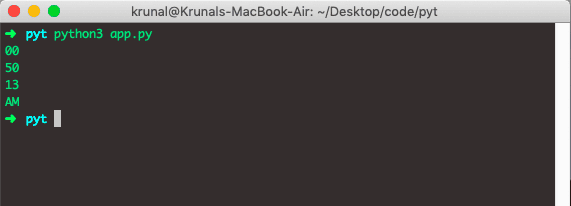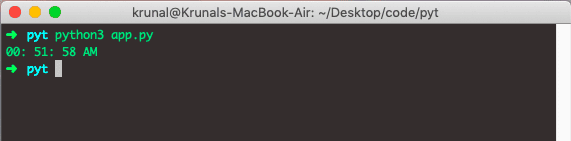# Python Time Format: The Complete Guide

In Python, date, time, and datetime classes provide some function to deal with the date, times, and time intervals.

## Python time format

To format time in Python, use the strftime() method. The strftime() is a built-in Python function that converts date and time objects to their string representation. Python datetime module provides different class methods to change the format of time.

The date and datetime objects are in Python, so when you manipulate them, you use objects, not string or timestamps.

If you want to know how to format the date in Python, check out the Python Date Format post.

We will use the datetime module’s datetime object and call the now() method. It will also return an object, and we will save it to the variable, and then we call the strftime() method on that object and pass the different abbreviations to that object.

## Python time abbreviation

%H – hour, using the 24-hour clock (00 to 23)

%I – hour, using the 12-hour clock (01 to 12)

%M – minute

%p – either am or pm according to a given time value

%r – time in the a.m. and p.m. notation

%R – time in 24-hour notation

%S – second

%T – current time, which is equal to %H:%M:%S

## Python strftime()

Python strftime() is a built-in module method that converts the tuple or struct_time representing time as returned by localtime() to the string specified by the format argument.

If t is not provided, the current time as returned by localtime() is used. Again, the format must be in the string.

Let’s see the following example.

```# app.py

from datetime import datetime

now = datetime.now()

print(now.strftime('%H'))
print(now.strftime('%M'))
print(now.strftime('%S'))
print(now.strftime('%p'))```

See the output.Let’s combine them to look like real-time.

```# app.py

from datetime import datetime

now = datetime.now()

print(now.strftime('%H: %M: %S %p'))```

See the outputThat’s it for this tutorial.

This site uses Akismet to reduce spam. Learn how your comment data is processed.